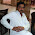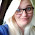## Friday, 9 August 2013

### Calculate Length of Service in Excel

If you are using Excel to store data about employees in a company, you may at some point need to calculate their length of service. Let’s say we want to return how many years and months an employee has worked for our company.

The DATEDIF function in Excel is used to calculate the difference between two dates. The difference can be returned as the number of years, months or days.

As we wish to return the number of years and months that they have worked we will concatenate two DATEDIF functions together.

## Calculate Length of Service Formula

Using the data in the spreadsheet below we can calculate the length of service for each employee in column E by using the following formula.

=DATEDIF(C2,D2,”y”)&” years, “ & DATEDIF(C2,D2,”ym”)&”months”

It is important that columns C and D are formatted as dates. If you receive an error for your formula this is a good place to check first for mistakes.

If you do not have an end date yet for the employee, the TODAY() function can be inserted into the formula in place of D2. This will calculate the length of service up to the current date.

## The DATEDIF Function

The DATEDIF function is not documented in Excel (for some strange reason) so when you type it into a cell no information is displayed.

Its syntax however is;

=DATEDIF(Start Date, End Date, Interval)

In our formula we wrote one that returned the number of year served, another for the number of months remaining after years is calculated and joined them together.

The & is also used to concatenate, or join, the years and months text into our answer.

If you like this check out these 5 awesome date functions in Excel.

### Watch the Video

Watch the

1.sie calulate length of service in excel download kese hoge

2.sie calulate length of service in excel download kese hoge

3.My formula is :

=DATEDIF(O2,AL\$1,"y")&" years, "&DATEDIF(O2,AL\$1,"ym")&" months, "&O2-DATE(YEAR(O2),MONTH(O2),1)&" days"

Where O2 is the hire date and AL\$1 is today()

The formula works, kinda. It isn't doing the math correctly. I have a person who started exactly a year ago and it is 2 weeks short...

4.i need to do from the hire date hrough this year, not todays date. what is that formula?

1.I have the same question! All I'm finding is "today" and "months" formulas. Just need formula to calculate YOS from hire date only... 01/11/1988 to the year 2019. No "start date" and "end date".

.